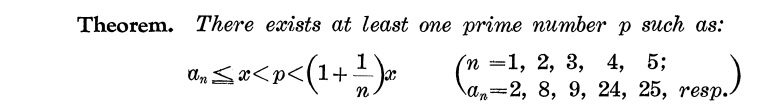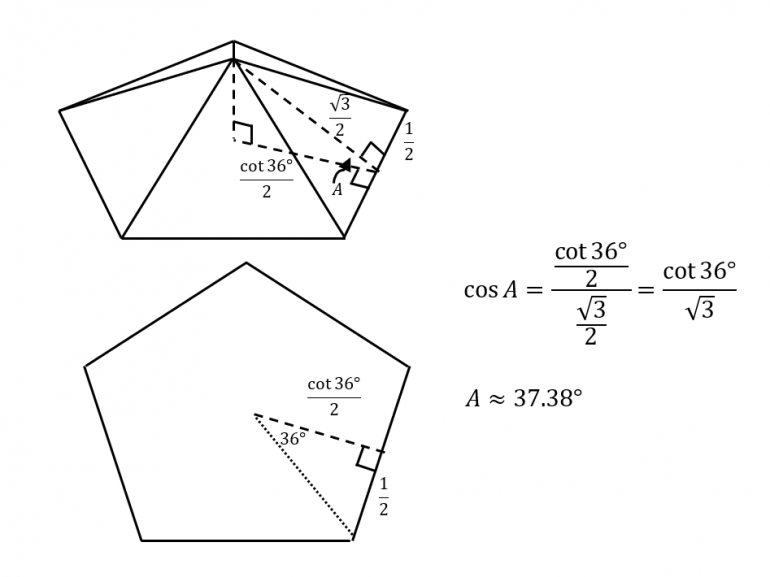## Poll

 I love math!18 votes (50%) Math is great.13 votes (36.11%) My religion is mathology.5 votes (13.88%) Women didn't speak to me until I was 30.2 votes (5.55%) Total eclipse reminder -- 04/08/202411 votes (30.55%) I steal cutlery from restaurants.3 votes (8.33%) I should just say what's on my mind.6 votes (16.66%) Who makes up these awful names for pandas?5 votes (13.88%) I like to touch my face.11 votes (30.55%) Pork chops and apple sauce.8 votes (22.22%)

36 members have voted

teliotJoined: Oct 19, 2009
• Posts: 2352
October 14th, 2021 at 12:24:56 PM permalink
Quote: ThatDonGuy

Quote: ThatDonGuy

Quote: teliot

For any integer N, consider the list of all primes that are in the half-open interval (N/2,N]. Call them p1, p2, p3 ... , pk. The question is, for which N is N!/(p1*p2*p3*...*pk) a perfect square? In other words, divide N! by all the primes greater than N/2 and less than or equal to N (the Bertrand's Postulate primes) and ask if what's leftover is a perfect square.

Any others? Infinitely many? This isn't in the OEIS (at least that I can find).

I wrote something that can calculate for larger numbers, and have not found any others for any N < 2000.

Update: no other solutions for N <= 3300. I stopped there as it is taking about 12 seconds to check each further number.

I would say this now constitutes an interesting open question in elementary number theory -- whether another such N exists ... such questions are hard to come by.
End of the world website: www.climatecasino.net
GMJoined: Jun 16, 2021
• Posts: 39
Thanks for this post from:October 14th, 2021 at 3:32:27 PM permalink
Quote: teliot

The question about N! not being a perfect square for N >= 2 leads to a question that I don't know the answer to. Here goes.

For any integer N, consider the list of all primes that are in the half-open interval (N/2,N]. Call them p1, p2, p3 ... , pk. The question is, for which N is N!/(p1*p2*p3*...*pk) a perfect square? In other words, divide N! by all the primes greater than N/2 and less than or equal to N (the Bertrand's Postulate primes) and ask if what's leftover is a perfect square.

Here are the five examples I found searching N <= 22 (the largest N for which Excel accurately computes N!).

With N = 6, then we divide 6! by 5 and 6!/5 = 144 is a perfect square.
With N = 7, then we divide 7! by 5*7 = 35 and get 7!/35 = 144, again a perfect square.
With N = 10, then we divide 10! by 7 (the only prime in the interval (5,10]) and get 10!/7 = 518400 = 720^2 is a perfect square.
With N = 11, then we divide 11! by 11*7 and again get 10!/7 = 518400 = 720^2.
With N = 20, then we divide 20! by 19*17*13*11 and get 52672757760000 = 7257600^2.

Any others? Infinitely many? This isn't in the OEIS (at least that I can find).

There is a paper by Jitsuro Nagura, which proves that for any real number x≥9, there exists a prime p such that x≤p<4x/3. If N≥35, we can apply this theorem with x=(N+1)/4 to obtain that there exists a prime p such that (N+1)/4≤p<(N+1)/3. Then p>N/4 clearly, and 3p<N+1, so 3p≤N, because 3p and N are integers, therefore p≤N/3.The only numbers among 1,2,...,N which are divisible by p are p, 2p and 3p, so N! is divisible by p^3 but not by p^4. After factoring out the primes between N and 2N, the resulting number will still be divisible by p^3, but not by p^4, so it cannot be a square.

This means that if N! divided by the product of primes in (N/2,N] is a square then N≤34. It is easy to check that the only solutions are N=2,3,6,7,10,11,20.
teliotJoined: Oct 19, 2009
• Posts: 2352
October 14th, 2021 at 6:11:50 PM permalink
Quote: GM

Quote: teliot

The question about N! not being a perfect square for N >= 2 leads to a question that I don't know the answer to. Here goes.

For any integer N, consider the list of all primes that are in the half-open interval (N/2,N]. Call them p1, p2, p3 ... , pk. The question is, for which N is N!/(p1*p2*p3*...*pk) a perfect square? In other words, divide N! by all the primes greater than N/2 and less than or equal to N (the Bertrand's Postulate primes) and ask if what's leftover is a perfect square.

Here are the five examples I found searching N <= 22 (the largest N for which Excel accurately computes N!).

With N = 6, then we divide 6! by 5 and 6!/5 = 144 is a perfect square.
With N = 7, then we divide 7! by 5*7 = 35 and get 7!/35 = 144, again a perfect square.
With N = 10, then we divide 10! by 7 (the only prime in the interval (5,10]) and get 10!/7 = 518400 = 720^2 is a perfect square.
With N = 11, then we divide 11! by 11*7 and again get 10!/7 = 518400 = 720^2.
With N = 20, then we divide 20! by 19*17*13*11 and get 52672757760000 = 7257600^2.

Any others? Infinitely many? This isn't in the OEIS (at least that I can find).

There is a paper by Jitsuro Nagura, which proves that for any real number x≥9, there exists a prime p such that x≤p<4x/3. If N≥35, we can apply this theorem with x=(N+1)/4 to obtain that there exists a prime p such that (N+1)/4≤p<(N+1)/3. Then p>N/4 clearly, and 3p<N+1, so 3p≤N, because 3p and N are integers, therefore p≤N/3.The only numbers among 1,2,...,N which are divisible by p are p, 2p and 3p, so N! is divisible by p^3 but not by p^4. After factoring out the primes between N and 2N, the resulting number will still be divisible by p^3, but not by p^4, so it cannot be a square.

This means that if N! divided by the product of primes in (N/2,N] is a square then N≤34. It is easy to check that the only solutions are N=2,3,6,7,10,11,20.

An elegant and simple argument. Many thanks!

This reminds me of a theorem I have long since forgotten, an improvement on Bertrand's Postulate that follows from the PNT, that for any e > 0 there is a positive integer N such that for all n > N, there exists a prime p with n <= p < (1+e)n. The result you quoted was this bound N being explicitly computed when e equals 1/3.

Again fabulous work.

By the way, I tried to look up your reference, Wikipedia pointed to this result by Nagura: there is always a prime between n and 6/5*n for n >= 25. I couldn't find the result in the paper you referenced. But your proof holds, we would just have to run the exhaustive search out a bit further.
Last edited by: teliot on Oct 14, 2021
End of the world website: www.climatecasino.net
ben771williamsJoined: Oct 11, 2021
• Posts: 21
October 15th, 2021 at 5:18:25 AM permalink
here's another puzzle that's worthy of a beer
GMJoined: Jun 16, 2021
• Posts: 39
Thanks for this post from:October 15th, 2021 at 5:45:00 AM permalink
Quote: teliot

By the way, I tried to look up your reference, Wikipedia pointed to this result by Nagura: there is always a prime between n and 6/5*n for n >= 25. I couldn't find the result in the paper you referenced. But your proof holds, we would just have to run the exhaustive search out a bit further.

There is a link on that page to download the whole paper, the statement of the theorem is at the bottom of the penultimate page.
teliotJoined: Oct 19, 2009
• Posts: 2352
October 15th, 2021 at 5:50:58 AM permalink
Quote: GM

Quote: teliot

By the way, I tried to look up your reference, Wikipedia pointed to this result by Nagura: there is always a prime between n and 6/5*n for n >= 25. I couldn't find the result in the paper you referenced. But your proof holds, we would just have to run the exhaustive search out a bit further.

There is a link on that page to download the whole paper, the statement of the theorem is at the bottom of the penultimate page.By the way, one consequence of an easy generalization of your argument is that for any integer k there exists an integer N such that for n > N there is a prime p so that p^k exactly divides n!.
Last edited by: teliot on Oct 15, 2021
End of the world website: www.climatecasino.net
GialmereJoined: Nov 26, 2018
• Posts: 2326
October 18th, 2021 at 7:48:42 AM permalinkConsider a pyramid with a base of a regular N-gon. All other sides are triangles and every edge is of unit length.

1) What is the maximum N?

2) What is the angle between the base and a side?

3) What is the angle between two adjacent sides?
Have you tried 22 tonight? I said 22.
acesideJoined: May 14, 2021
• Posts: 136
Thanks for this post from:October 18th, 2021 at 5:59:24 PM permalink
Quote: GialmereConsider a pyramid with a base of a regular N-gon. All other sides are triangles and every edge is of unit length.

1) What is the maximum N?

2) What is the angle between the base and a side?

3) What is the angle between two adjacent sides?

Apparently N=5, because when N=6, that pyramid will be flat.
WizardJoined: Oct 14, 2009
• Posts: 24233
Thanks for this post from:October 18th, 2021 at 6:56:26 PM permalink

1. 5. At six, the pyramid would be flat. I'm not sure if that counts as a pyramid.
2. I'll bother to answer that if you confirm my answer of 5.
3. Based on a pentagonal base, 108.

I have a feeling I am not understanding the question correctly.
It's not whether you win or lose; it's whether or not you had a good bet.
ChesterDogJoined: Jul 26, 2010
• Posts: 1026
Thanks for this post from:October 18th, 2021 at 7:09:47 PM permalink
Quote: GialmereConsider a pyramid with a base of a regular N-gon. All other sides are triangles and every edge is of unit length.

...
2) What is the angle between the base and a side?

...